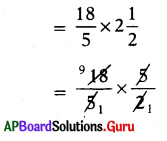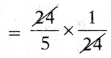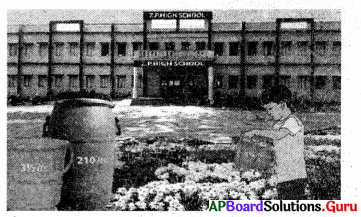SCERT AP 7th Class Maths Solutions Pdf Chapter 2 Fractions, Decimals and Rational Numbers Ex 2.1 Textbook Exercise Questions and Answers.

AP State Syllabus 7th Class Maths Solutions 2nd Lesson Fractions, Decimals and Rational Numbers Exercise 2.1

Question 1.
In Jagananna Gorumudda (MDM) scheme each student got $$\frac{3}{20}$$ kg. rice per day, find the weight of the rice required for 60 students in a class per day.
Given quantity of rice each student get per day = $$\frac{3}{20}$$ kg.
Quantity of rice 60 students get per day
= $$\frac{3}{20} \times \frac{60}{1}$$
= $$\frac{3 \times 60}{20 \times 1}$$
= $$\frac{180}{20}$$ = 9

∴ Quantity of rice required for 60 students per day = 9 kg.

Question 2.
What is a perimeter of an equilateral triangle, if each*side of a triangle is 5$$\frac{3}{10}$$cm?
Let the side of an equilateral triangle be x cm.
Perimeter of an equilateral triangle = 3x.
= 3 × 5 $${30}{10}$$
= $$\frac{3}{1} \times \frac{53}{10}$$
= $$\frac{3 \times 53}{1 \times 10}$$

∴ Perimeter of triangle = $$\frac{159}{10}$$ or 15$$\frac{9}{10}$$ cmQuestion 3.
Surya can walk $$\frac{18}{5}$$kilo meters in an hour. How much distance can he walk in 2$$\frac{1}{2}$$ hours ?
Given, distance walked by Surya in 1 hour = $$\frac{18}{5}$$km.
Distance walked by Surya in 2$$\frac{1}{2}$$hours∴ Distance walked in 2$$\frac{1}{2}$$hours
= $$\frac{9}{1}$$ = 9 km

Question 4.
If the length and breadth of a rectan-gular garden are $$\frac{27}{2}$$m and $$\frac{15}{2}$$m respectively, then find the area of the garden.
Given, the length of a garden = $$\frac{27}{2}$$m.
breadth of a garden = $$\frac{15}{2}$$m.
Area of rectangular garden = length × breadth
= $$\frac{27}{2} \times \frac{15}{2}$$
= $$\frac{27 \times 15}{2 \times 2}$$

∴ Area of the garden = $$\frac{405}{4}$$ or 101$$\frac{1}{4}$$

Question 5.
Gopal bought 3$$\frac{1}{2}$$kg. of potatoes in the market. If he paid ?84, then find the cost of 1 kg. of potatoes.
Given, cost of 3$$\frac{1}{2}$$ kgs of potatoes = ₹ 84
Cost of 1 kg of potatoes = 84 ÷ 3$$\frac{1}{2}$$
= 84 ÷ $$\frac{7}{2}$$
= $$\frac{84}{1} \times \frac{2}{7}$$

∴ Cost of one kg of potatoes = $$\frac{168}{7}$$
= ₹ 24

Question 6.
A car travelled 225 km. in 4 $$\frac{1}{2}$$ hours with uniform speed. Find the distance travelled in 1 hour.
Given, distance travelled in 4$$\frac{1}{2}$$ hours = 225 km
Distance travelled in 1 hour
= 225 ÷ 4$$\frac{1}{2}$$
= 225 ÷ $$\frac{9}{2}$$
= $$\frac{225}{1} \times \frac{2}{9}$$
= $$\frac{225 \times 2}{1 \times 9}$$

∴ Distance travelled by car in 1 hour
= $$\frac{450}{2}$$ = 50 km

Question 7.
If 24 students share 4$$\frac{4}{5}$$kg. of cake, then how much cake does each one get?
Given, quantity of cake shared by 24 students = 4$$\frac{4}{5}$$kg

Quantity of cake each one got
= 4$$\frac{4}{5}$$ ÷ 24 .
= $$\frac{24}{5} \div \frac{24}{1}$$∴ Quantity of cake each one got = $$\frac{1}{5}$$kg (or) 0.2 kg (or) 200 gm.Question 8.
A drum contains 210 l of water. How many times does the boy get the water for watering die plants with 3$$\frac{1}{2}$$ l of full bucket from the drum ?Capacity of bucket = 3$$\frac{1}{2}$$ l
Number of buckets of water the boy get = 210 ÷ 3$$\frac{1}{2}$$
= 210 ÷ $$\frac{7}{2}$$
= $$\frac{210}{1} \times \frac{2}{7}$$
= $$\frac{420}{7}$$ = 60